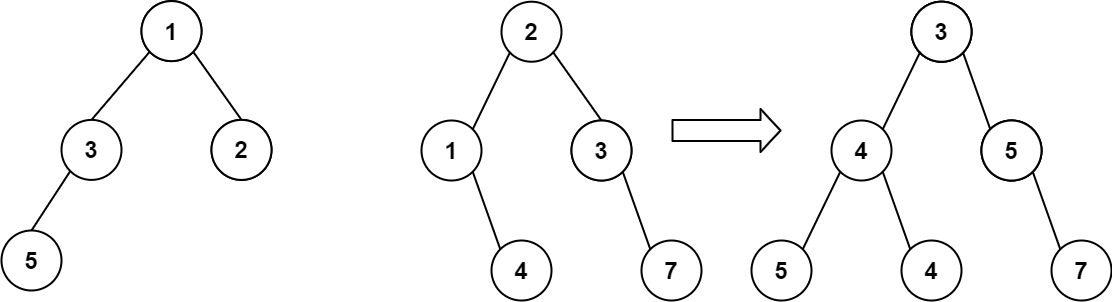## Algorithm

Problem Name: 617. Merge Two Binary Trees

You are given two binary trees `root1` and `root2`.

Imagine that when you put one of them to cover the other, some nodes of the two trees are overlapped while the others are not. You need to merge the two trees into a new binary tree. The merge rule is that if two nodes overlap, then sum node values up as the new value of the merged node. Otherwise, the NOT null node will be used as the node of the new tree.

Return the merged tree.

Note: The merging process must start from the root nodes of both trees.

Example 1:```Input: root1 = [1,3,2,5], root2 = [2,1,3,null,4,null,7]
Output: [3,4,5,5,4,null,7]
```

Example 2:

```Input: root1 = , root2 = [1,2]
Output: [2,2]
```

Constraints:

• The number of nodes in both trees is in the range `[0, 2000]`.
• `-104 <= Node.val <= 104`

## Code Examples

### #1 Code Example with Java Programming

```Code - Java Programming```

``````
class Solution {
public TreeNode mergeTrees(TreeNode root1, TreeNode root2) {
if (root1 == null && root2 == null) {
return null;
}
int value = (root1 == null ? 0 : root1.val) + (root2 == null ? 0 : root2.val);
TreeNode root = new TreeNode(value);
root.left = mergeTrees((root1 == null ? null : root1.left), (root2 == null ? null : root2.left));
root.right = mergeTrees((root1 == null ? null : root1.right), (root2 == null ? null : root2.right));
return root;
}
}
``````
Copy The Code &

Input

cmd
root1 = [1,3,2,5], root2 = [2,1,3,null,4,null,7]

Output

cmd
[3,4,5,5,4,null,7]

### #2 Code Example with Python Programming

```Code - Python Programming```

``````
class Solution:
def mergeTrees(self, t1, t2):
"""
:type t1: TreeNode
:type t2: TreeNode
:rtype: TreeNode
"""
if t1 and t2:
root, root.left, root.right = TreeNode(t1.val + t2.val), self.mergeTrees(t1.left, t2.left), self.mergeTrees(t1.right, t2.right)
return root
else: return t1 or t2
``````
Copy The Code &

Input

cmd
root1 = [1,3,2,5], root2 = [2,1,3,null,4,null,7]

Output

cmd
[3,4,5,5,4,null,7]

### #3 Code Example with C# Programming

```Code - C# Programming```

``````
namespace LeetCode
{
public class _0617_MergeTwoBinaryTrees
{
public TreeNode MergeTrees(TreeNode t1, TreeNode t2)
{
if (t1 == null) return t2;
if (t2 == null) return t1;

var newNode = new TreeNode(t1.val + t2.val);
newNode.left = MergeTrees(t1.left, t2.left);
newNode.right = MergeTrees(t1.right, t2.right);
return newNode;
}
}
}
``````
Copy The Code &

Input

cmd
root1 = , root2 = [1,2]

Output

cmd
[2,2]